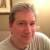# 10 Extremely Useful .NET Extension Methods

### Since Extension methods were introduced in C# 3.0, I have constantly been looking for ways to extend classes to ease my coding pain. Here are my 10 favorite extension methods.

Written by Jonathan "JD" Danylko • Last Updated: • Develop •Have you ever had a class that just doesn't do enough for you? Yeah, same here.

I have always wanted to add more onto a DateTime class, but I just couldn't. That is, until extension methods were introduced in C# 3.0.

Now, I have to admit...I'm an extension method junkie. I have a folder in my library called Extensions and it has a good number of classes that I use every day to make my life easier.

## What are they?

Extension Methods allow you to create new functionality for existing types without modifying the actual type itself. As I mentioned before, the characteristics of an extension method are as follows:

• The class has to be static
• The method has to be static
• The method's first parameter in the signature must have the "this" declared.
• The scope has to be public

All you are doing is attaching methods to existing types. If you don't like the way a method works on the String class, write an extension method for it.

Ok, so on with the top 10 extension methods that I love.

NOTE: These extensions are running on production systems so they've been tested. :-)

1. ToFileSize - Type: Long

This extension method was necessary because it was easier to read the number, but then I thought, I'm reading file sizes. Why not just convert it into formatted number with the correct size metric.
```public static class MiscExtensions
{
public static string ToFileSize(this long size)
{
if (size < 1024) { return (size).ToString("F0") + " bytes"; }
if (size < Math.Pow(1024, 2)) { return (size/1024).ToString("F0") + "KB"; }
if (size < Math.Pow(1024, 3)) { return (size/Math.Pow(1024, 2)).ToString("F0") + "MB"; }
if (size < Math.Pow(1024, 4)) { return (size/Math.Pow(1024, 3)).ToString("F0") + "GB"; }
if (size < Math.Pow(1024, 5)) { return (size/Math.Pow(1024, 4)).ToString("F0") + "TB"; }
if (size < Math.Pow(1024, 6)) { return (size/Math.Pow(1024, 5)).ToString("F0") + "PB"; }
return (size/Math.Pow(1024, 6)).ToString("F0") + "EB";
}
}```
2. ToXmlDocument()/ToXDocument() - Type: XDocument or XmlDocument

I can't tell you how many times I've need to convert an XmlDocument into an XDocument and vice-versa to use LINQ. These handy extension methods will save you a load of time.
```public static class XmlDocumentExtensions
{
public static XmlDocument ToXmlDocument(this XDocument xDocument)
{
var xmlDocument = new XmlDocument();
{
}
return xmlDocument;
}
public static XDocument ToXDocument(this XmlDocument xmlDocument)
{
{
}
}
public static XmlDocument ToXmlDocument(this XElement xElement)
{
var sb = new StringBuilder();
var xws = new XmlWriterSettings {OmitXmlDeclaration = true, Indent = false};
using (var xw = XmlWriter.Create(sb, xws))
{
xElement.WriteTo(xw);
}
var doc = new XmlDocument();
return doc;
}
public static Stream ToMemoryStream(this XmlDocument doc)
{
var xmlStream = new MemoryStream();
doc.Save(xmlStream);
xmlStream.Flush();//Adjust this if you want read your data
xmlStream.Position = 0;
return xmlStream;
}
}```
3. RemoveLast()/RemoveLastCharacter()/RemoveFirst()/RemoveFirstCharacter() - Type: String

These may seem trivial, but in the heat of string manipulation, it's so much easier to "remove the last character" from the string and get on with your life.
```public static string RemoveLastCharacter(this String instr)
{
return instr.Substring(0, instr.Length - 1);
}
public static string RemoveLast(this String instr, int number)
{
return instr.Substring(0, instr.Length - number);
}
public static string RemoveFirstCharacter(this String instr)
{
return instr.Substring(1);
}
public static string RemoveFirst(this String instr, int number)
{
return instr.Substring(number);
}
```
4. Between() - Type: DateTime

Check to see if a date is between two dates. 'Nuff said.
```public static bool Between(this DateTime dt, DateTime rangeBeg, DateTime rangeEnd)
{
return dt.Ticks >= rangeBeg.Ticks && dt.Ticks <= rangeEnd.Ticks;
}```
5. CalculateAge() - Type: DateTime

Figure out how old something (or someone) is.
```public static int CalculateAge(this DateTime dateTime)
{
var age = DateTime.Now.Year - dateTime.Year;
if (DateTime.Now < dateTime.AddYears(age))
age--;
return age;
}```
6. ToReadableTime() - Type: DateTime

Based on the time, it will display a readable sentence as to when that time happened (i.e. 'One second ago' or '2 months ago')
```public static string ToReadableTime(this DateTime value)
{
var ts = new TimeSpan(DateTime.UtcNow.Ticks - value.Ticks);
double delta = ts.TotalSeconds;
if (delta < 60)
{
return ts.Seconds == 1 ? "one second ago" : ts.Seconds + " seconds ago";
}
if (delta < 120)
{
return "a minute ago";
}
if (delta < 2700) // 45 * 60
{
return ts.Minutes + " minutes ago";
}
if (delta < 5400) // 90 * 60
{
return "an hour ago";
}
if (delta < 86400) // 24 * 60 * 60
{
return ts.Hours + " hours ago";
}
if (delta < 172800) // 48 * 60 * 60
{
return "yesterday";
}
if (delta < 2592000) // 30 * 24 * 60 * 60
{
return ts.Days + " days ago";
}
if (delta < 31104000) // 12 * 30 * 24 * 60 * 60
{
int months = Convert.ToInt32(Math.Floor((double)ts.Days / 30));
return months <= 1 ? "one month ago" : months + " months ago";
}
var years = Convert.ToInt32(Math.Floor((double)ts.Days / 365));
return years <= 1 ? "one year ago" : years + " years ago";
}
```
7. WorkingDay()/IsWeekend()/NextWorkday() - Type: DateTime

Determine if the date is a working day, weekend, or determine the next workday coming up.
```public static bool WorkingDay(this DateTime date)
{
return date.DayOfWeek != DayOfWeek.Saturday && date.DayOfWeek != DayOfWeek.Sunday;
}
public static bool IsWeekend(this DateTime date)
{
return date.DayOfWeek == DayOfWeek.Saturday || date.DayOfWeek == DayOfWeek.Sunday;
}
public static DateTime NextWorkday(this DateTime date)
{
var nextDay = date.AddDays(1);
while (!nextDay.WorkingDay())
{
}
return nextDay;
}
```
8. Next() - Type: DateTime

Determine the Next date by passing in a DayOfWeek (i.e. From this date, when is the next Tuesday?)
```public static DateTime Next(this DateTime current, DayOfWeek dayOfWeek)
{
int offsetDays = dayOfWeek - current.DayOfWeek;
if (offsetDays <= 0)
{
offsetDays += 7;
}
DateTime result = current.AddDays(offsetDays);
return result;
}
```
9. str.ToStream()/stream.ToString()/CopyTo() - Type: String/Stream

If you want to take a large string and convert it to a Stream or vice-versa, here are three extension methods that make this the easiest way to manipulate streams.
```public static Stream ToStream(this string str)
{
byte[] byteArray = Encoding.UTF8.GetBytes(str);
//byte[] byteArray = Encoding.ASCII.GetBytes(str);
return new MemoryStream(byteArray);
}
public static string ToString(this Stream stream)
{
}
/// <summary>
/// Copy from one stream to another.
/// Example:
/// using(var stream = response.GetResponseStream())
/// using(var ms = new MemoryStream())
/// {
///     stream.CopyTo(ms);
///      // Do something with copied data
/// }
/// </summary>
/// <param name="fromStream">From stream.</param>
/// <param name="toStream">To stream.</param>
public static void CopyTo(this Stream fromStream, Stream toStream)
{
if (fromStream == null)
throw new ArgumentNullException("fromStream");
if (toStream == null)
throw new ArgumentNullException("toStream");
var bytes = new byte;
while ((dataRead = fromStream.Read(bytes, 0, bytes.Length)) > 0)
}
```
10. Has<T>()/Is<T>()/Add<T>()/Remove<T>() - Type: Enum

This is a great extension when an enumerated type are flags instead of full items. One example is to have an integer in a table that converts to Enumerated Flag types. This is where these extensions come in handy (I may write about this for Friday).
```public static bool Has<T>(this System.Enum type, T value)
{
try
{
return (((int)(object)type & (int)(object)value) == (int)(object)value);
}
catch
{
return false;
}
}
public static bool Is<T>(this System.Enum type, T value)
{
try
{
return (int)(object)type == (int)(object)value;
}
catch
{
return false;
}
}
public static T Add<T>(this System.Enum type, T value)
{
try
{
return (T)(object)(((int)(object)type | (int)(object)value));
}
catch (Exception ex)
{
throw new ArgumentException(
string.Format(
"Could not append value from enumerated type '{0}'.",
typeof(T).Name
), ex);
}
}
public static T Remove<T>(this System.Enum type, T value)
{
try
{
return (T)(object)(((int)(object)type & ~(int)(object)value));
}
catch (Exception ex)
{
throw new ArgumentException(
string.Format(
"Could not remove value from enumerated type '{0}'.",
typeof(T).Name
), ex);
}
}
```

## Conclusion

In my eyes, extension methods are very powerful and can be extended until your heart's content. I hope these extension methods get you thinking about how to extend existing classes to provide even more functionality.

Have you created any extension methods? What helpful methods did you create? Post you comments below.Jonathan Danylko is a web architect and entrepreneur who's been programming for over 25 years. He's developed websites for small, medium, and Fortune 500 companies since 1996.

He currently works at Insight Enterprises as a Principal Software Engineer.

When asked what he likes to do in his spare time, he replies, "I like to write and I like to code. I also like to write about code."